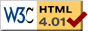### Journée-séminaire de combinatoire

#### (équipe CALIN du LIPN, université Paris-Nord, Villetaneuse)

Le 26 mai 2015 à 14h00 en B107, Louis Dumont nous parlera de : Efficient Algebraic Diagonals and Walks

Résumé : The diagonal of a multivariate power series F is the univariate power series Diag F generated by the diagonal terms of F. Diagonals form an important class of power series; they occur frequently in number theory, theoretical physics and enumerative combinatorics. We study algorithmic questions related to diagonals in the case where F is the Taylor expansion of a bivariate rational function. It is classical that in this case Diag F is an algebraic function. We propose an algorithm that computes an annihilating polynomial for Diag F. Generically, it is its minimal polynomial and is obtained in time quasi-linear in its size. We show that this minimal polynomial has an exponential size with respect to the degree of the input rational function. Throughout the talk, we use a common problem of counting certain lattice walks to illustrate the capacities and limits of our tools.

 Dernière modification : Monday 24 January 2022Contact pour cette page : Cyril.Banderier at lipn.univ-paris13.fr